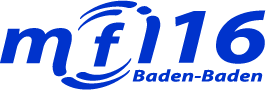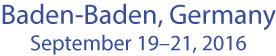T8 Proactive Optimal Control to Infer Information Faster

Length: 6 hours

Intended Audience: A short introduction to Euler-Lagrange equation, least squares method and regulators will be provided, so basic understanding of university course on functions of several variables and their differentials would be enough. Comprehending that the derivative of a function equals to zero at its maximum and minimum values means a sufficient background for this tutorial.

Description: Assume that we a have a robot and we know what force is applied to the mass center of the robot. Our inputs are the velocity of the robot and the known control force signal. We know that transient signals can tell us about the mass and friction coefficient based on boundary conditions of Newton’s second law. Putting it simpler, the start of the any transient process after switching on a control force tells us what the inertia of the robot is and the end time series provide information about steady state, which explains us, what the friction coefficient is. However, how to incorporate both start and end time series in a single inference step when mass and friction are changing in time? This tutorial answers this question. Complicating it even further, we will learn on how to extend this approach to detect not only smooth, but also abrupt (and relatively rare) changes. Recent paper of Entropy journal “Simultaneous State and Parameter Estimation Using Maximum Relative Entropy with Nonhomogenous Differential Equation Constraints” (at http://www.mdpi.com/1099-4300/16/9/4974/pdf) shows the derivation. We will reiterate whole derivation by putting emphasis on how to implement it practically.

Prerequisites: Prerequisites are Wolfram Mathematica (optional), Matlab (optional) or any C-language tool (optional) to provide the mathematical structure and increase understanding on how to write highspeed code or script.

Presenter: Renaldas Urniezius

Renaldas Urniezius received his B.Sc. and M.Sc. (with honors) degrees in the Electrical Engineering, Ph.D. in the Electronics Engineering from Kaunas University of Technology. He has been IEEE member since 2006 and currently belongs to Signal Processing and Robotics Societies. His research interests are process analysis and modeling, optimal control and Pontryagin's principle, sensor fusion and vision analysis applications in electromechanical systems, synthesis and research in foundations of inference and machine learning methods, optimal resource allocation using variational programming, optimal control of bioprocesses.